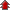Home Board Paper Solutions Text Book Solutions Articles NCERT Exemplar Solutions

# Electromagnetic Waves### Exercise 1

•  Q1 ">Figure 8.6 shows a capacitor made of two circular plates each of radius 12 cm, and separated by 5.0 cm. The capacitor is being charged by an external source (not shown in the figure). The charging current is constant and equal to 0.15 A.   (a) Calculate the capacitance and the rate of charge of potential difference between the plates.   (b) Obtain the displacement current across the plates.   (c) Is Kirchhoff’s first rule (junction rule) valid at each plate of the capacitor? Explain.Q3 What physical quantity is the same for X-rays of wavelength 10−10 m, red light of wavelength 6800 A and radiowaves of wavelength 500 m? Q4 A plane electromagnetic wave travels in vacuum along z-direction. What can you say about the directions of its electric and magnetic field vectors? If the frequency of the wave is 30 MHz, what is its wavelength? Q5 A radio can tune in to any station in the 7.5 MHz to 12 MHz band. What is the corresponding wavelength band? Q6 A charged particle oscillates about its mean equilibrium position with a frequency of 109 Hz. What is the frequency of the electromagnetic waves produced by the oscillator? Q7 The amplitude of the magnetic field part of a harmonic electromagnetic wave in vacuum is B0 = 510 nT. What is the amplitude of the electric field part of the wave? Q15 Answer the following questions: (a) Long distance radio broadcasts use short-wave bands. Why? (b) It is necessary to use satellites for long distance TV transmission. Why? (c) Optical and radio telescopes are built on the ground but X-ray astronomy is possible only from satellites orbiting the earth. Why? (d) The small ozone layer on top of the stratosphere is crucial for human survival. Why? (e) If the earth did not have an atmosphere, would its average surface temperature be higher or lower than what it is now? (f) Some scientists have predicted that a global nuclear war on the earth would be followed by a severe ‘nuclear winter’ with a devastating effect on life on earth. What might be the basis of this prediction?
• NCERT Chapter